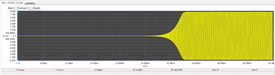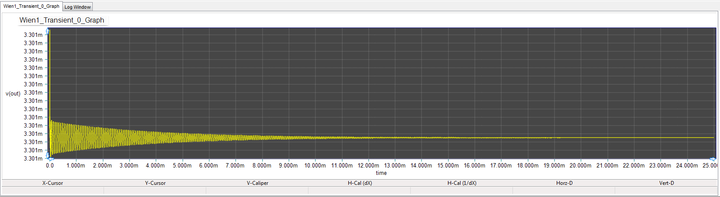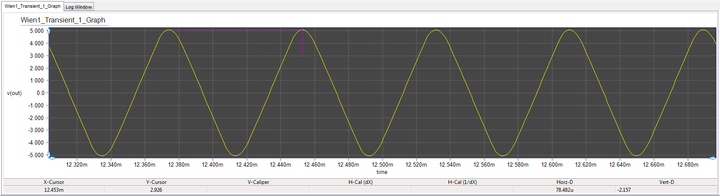Analog Tutorial Lesson 9: Designing a Wien Bridge Oscillator

 Tutorial Project: Designing a Wien Bridge OscillatorObjective: In this project, you will design and realize a Wien bridge oscillator and analyze its operation. Concepts/Features: Operational Amplifier Wien Bridge Oscillation Frequency Barkhausen Criterion Positive Feedback Minimum Version Required: All versions

What You Will Learn

In this tutorial you will build a Wien Bridge Oscillator using an LM741 Op-Amp and will analyze its oscillatory behavior. The primary objective of this tutorial lesson is to underline the challenges of analyzing oscillator circuits.

Designing a Wien Bridge Oscillator

The following is a list of parts needed for this part of the tutorial lesson:

Part Name Part Type Part Value
VCC DC Voltage Source 15V
VEE DC Voltage Source -15V
R1 - R3 Resistor 10k
R4 Resistor 20k
C1 - C2 Capacitor 1n
X1 LM741 Op-Amp Defaults

The Wien Bridge Oscillator comprises an Op-Amp, four resistors and two capacitors. The oscillator can also be viewed as a positive gain amplifier combined with a bandpass filter that provides positive feedback. At the resonant frequency, the reactance of the series R2–C2 arm will be an exact multiple of the shunt R1–C1 arm. If the two R3 and R4 arms are adjusted to the same ratio, then the bridge is balanced. These conditions can be written as:

$\omega^2 = \frac {1}{R_1 R_2 C_1 C_2}$

and

$\frac {C_1}{C_2} = \frac {R_4}{R_3} - \frac {R_2}{R_1}$

This can be further simplified by setting R1 = R2 = R and C1 = C2 = C. In that case, setting R4 = 2 R3 will satisfy the above conditions and will lead to the following simple equation for the oscillation frequency:

$f_o = \frac {1}{2 \pi RC}$

For this project, you will initially choose R1 = R2 = 10k, R3 = 10k, R3 = 2 R3 = 20k, and C1 = C3 = 100pF. Place and connect all the parts as shown in the above figure. Place a voltage probe marker at the output of the Op-Amp. The oscillation frequency of this circuit must be fo = 1 / (2π . 1e+4 . 1e-9) = 15.9kHz.

Testing the Wien Bridge Oscillator

Run a Transient Test of this circuit with start and stop times set to 0 and 25ms and a Step Ceiling of 1μs. You will get a graph like the one shown below.The output voltage of the Wien Bridge Oscillator when R4 = 20k.

The circuit is certainly not oscillating in a sustainable way as the output voltage fluctuates around 3.3mV. Next, change the value of R4 to 21k and run a new Transient Test. This time you will get a fairly large oscillation between -5V and +5V as show below.The output voltage of the Wien Bridge Oscillator when R4 = 21k.

In order to calculate the oscillation frequency, first zoom in the graph window by clicking the "Zoom In" button of the Graph Toolbar. Then use the "Delta Tool" of the Graph Toolbar to measure the distance between two successive peaks of the sinusoidal signal. The period is about 78.5μs, which gives an oscillation frequency of 12.75kHz.Measuring the signal period on a close-up of the output voltage of the Wien Bridge Oscillator when R4 = 21k.

Next, reduce the value of R4 further to 20.25k and run a new Transient Test again, but this time with a stop time of 50ms. You will notice that the sustainable oscillations start at much later time around 30ms with a reduced peak-to-peak level. If you zoom in the graph and measure the signal period, you will note that it has reduced to 71.5μs, which gives an oscillation frequency of about 14kHz, fairly close to the design frequency.The output voltage of the Wien Bridge Oscillator when R4 = 20.25k.

Analyzing the Wien Bridge Oscillator and Explaining Its Behavior

The above behavior of the circuit can be explain using the feedback theory. The Wien Bridge Oscillator is indeed an non-inverting Op-Amp amplifier with positive feedback provided using two series and shunt RC impedances:

$Z_1(s) = \frac {R . \frac{1}{sC}}{R + \frac{1}{sC}}$

$Z_2(s) = R + \frac{1}{sC}$

The gain of the non-inverting amplifier is given by:

$A = 1 + \frac{R_4}{R_3}$

The feedback gain can be found from the voltage divider:

$\beta (s) = \frac{Z_1(s)}{Z_1(s) + Z_2(s)} = \frac {sRC} {s^2 R^2 C^2 + 3sRC + 1}$

The Barkhausen criterion for oscillation requires that T(s) = Aβ(s) ≥ 1. Substituting s = jω, one can write:

$T(j\omega) = A \beta(j\omega) = \frac {j\omega RC} {(1-\omega ^2 R^2 C^2)+ 3j\omega RC}$

A zero phase shift can be achieved only if

$1-\omega ^2 R^2 C^2 = 0$

which lead to the oscillation frequency ωo = 1/(RC). This further simplifies the Barkhausen criterion to

$T(j\omega) = \frac {A}{3} \ge 1$

which means that A = 1 + R4/R3 ≥ 3 or R4 ≥ 2R3. This shows that setting R4 = 2R3 provides the threshold of oscillation. For R4 > 2R3, positive feedback causes the oscillations to grow indefinitely.